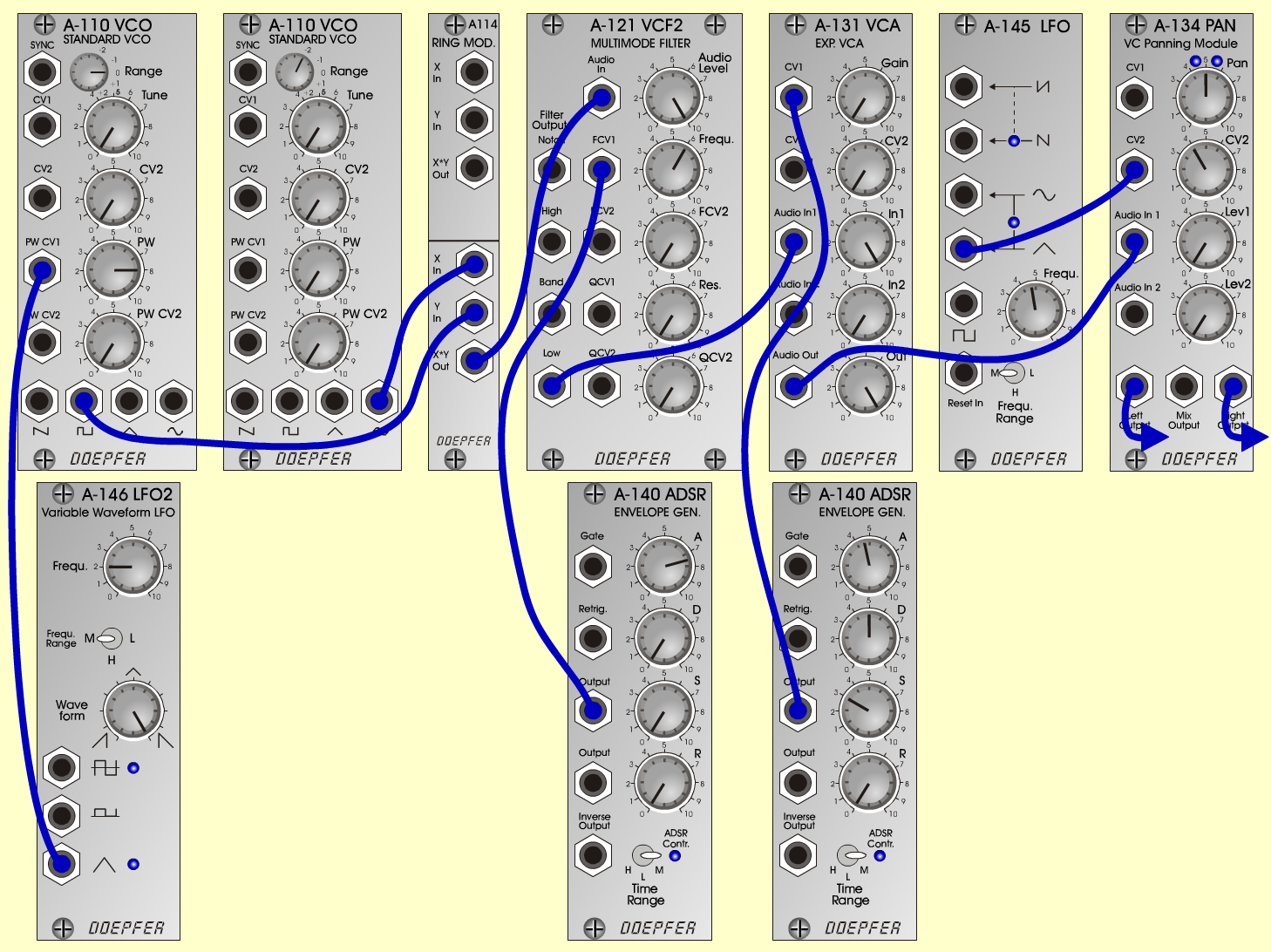DimorphopanS y n t hD o e p f e r A - 100 Connections: Settings: A-110/1 (Sine) <=> A-114 (X In) A-110/2 (Pulse) <=> A-114 (Y In) A-114 (Out) <=> A-121 (Audio In) A-121 (Low) <=> A-131 (Audio In) A-131 (Audio Out) <=> A-134 (Audio In 1) A-134 (Left-/Right-Out) <=> Stereo-Amplifier A-145 (Triangle) <=> A-134 (CV 2) KB (Gate) <=> A-145 (Reset In) KB (CV) <=> A-110/2 (CV 1) A-146 (Triangle) <=> A-110/2 (PW CV1) 140/1 (Output) <=> A-121 (FCV 1) A-140/2 (Output) <=> A-131 (CV 1) A-110/1 (Range = -2, Tune = 0) A-110/2 (PW = 8) A-121 (Audio Level = 10, Freq = 6, Res = 0) A-131 (Gain = 0, Audio In 1 = 10, Audio Out = 10) A-134 (Pan = 5, CV 2 = 4, Lev 1 = 10, Lev 2 = 10) A-145 (Frq = 4.7, Range = M) A-146 (Freq = 2, Wave Form = 10, Range = M) A-140/1 (A = 7.5, D = 0, S = 0, R = 0, Range = M) A-140/2 (A = 4.6, D = 5, S = 3, R = 0, Range = M) Josef MuellerSound samples Dimorphopan (130 Hz) Dimorphopan (261 Hz) Dimorphopan (523 Hz)